# Programmatic Access

In the Wolfram Language, Databin["ID"] represents a databin:

 In:=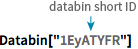X Databin["1EyATYFR"]
 Out=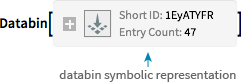Provided that your Wolfram Language system is connected to the cloud, you can access any Databin[...] for which you have permission.

Plot the contents of a databin against time:

 In:=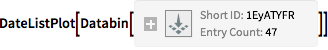X DateListPlot[Databin["1EyATYFR"]]
 Out=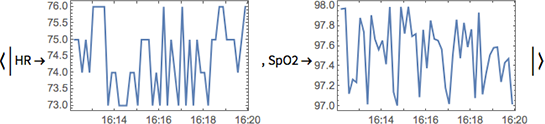TimeSeries[databin] gives a symbolic representation of the time series of values in
a databin:

 In:=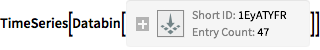X TimeSeries[Databin["1EyATYFR"]]
 Out=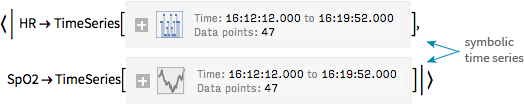QUICK REFERENCE: Time Series Analysis »

(Use TimeSeries when "points should be joined up"; EventSeries when each point is a separate event.)

Values[databin] gives a list of databin values, without timestamps:

 In:=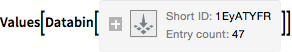X Values[Databin["1EyATYFR"]]
 Out=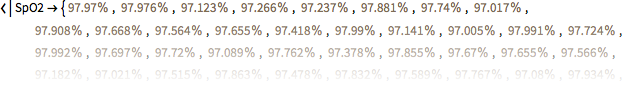Other databin functions:

 In:=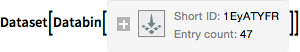X Dataset[Databin["1EyATYFR"]]
 Out=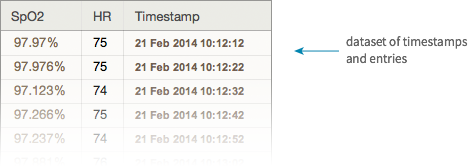In:=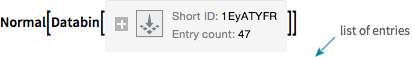X Normal[Databin["1EyATYFR"]]
 Out=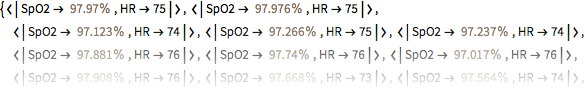In:=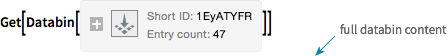X Get[Databin["1EyATYFR"]]
 Out=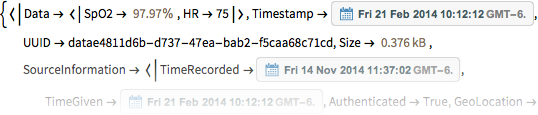Databin["ID"]["property"] gives a particular property of a databin:

 In:=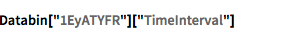X Databin["1EyATYFR"]["TimeInterval"]
 Out=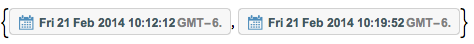Databin["ID"]["FullEntries"] gives all data and metadata for each entry in a databin.

QUICK REFERENCE: Databin Elements »